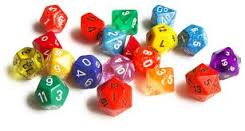# An $N$-sided dieJorge has an $N$-sided fair die and wonders how many times he would need to roll it until he has rolled all the numbers from $1$ to $N$ (in any order).

He does a quick calculation and discovers that the expected value for the number of rolls is 91 when rounding to the nearest integer.

How many sides does his die have?

Clarification: One distinct number from $1$ to $N$ is printed on each face of the die.

###### Image credit: ravnerdwars.info

Other Expected Value Quizzes

×